# Basic Arithmetic : Solving Linear Equations with Fractions

## Example Questions

### Example Question #1 : Solving Linear Equations With Fractions

Solve for x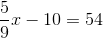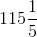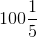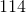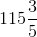Explanation:

Start by adding 10 to both sides.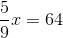Multiply both side by 9 to get rid of the fraction.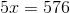Divide by 5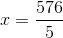Since all the answer choices have mixed fractions, you will also need to reduce down to a mixed fraction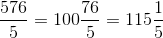### Example Question #11 : Linear Equations With Fractions

Solve for.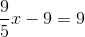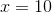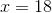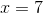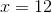Explanation:

Add both sides by 9 to isolate the x on one side.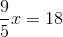Multiply both sides by 5.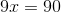Divide boths ides b 9.### Example Question #1 : Solving Linear Equations With Fractions

Solve for.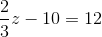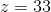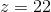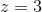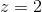Explanation:

First, add 10 to both sides so the term with "z" is isolated on one side.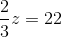To get rid of the fraction, multiply both sides by 3.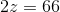Divide by 2.### Example Question #2 : Solving Linear Equations With Fractions

Solve for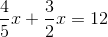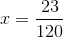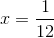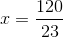Explanation:

Start by adding the terms withtogether. Find the least common denominator for the two fractions.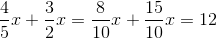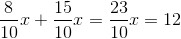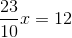Now, multiply both sides by 10.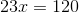Then divide both sides by 23.### Example Question #1 : Solving Linear Equations With Fractions

Solve for.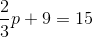Explanation:

Start by adding 9 to both sides.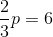Next, multiply both sides by 3.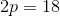Finally, divide both sides by 2.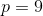### Example Question #1 : Solving Linear Equations With Fractions

Solve for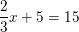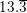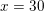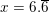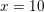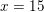Explanation:

When we are solving equations, we must always remember that what's on the left side equals the right side. Therefore, any changes that we make to one side of the equation, we must make to the other side so that the equation stays equal and balanced. When solving single-variable equations, we try to isolate the variable on one side so that we can get a number which it's equal to on the other side. Therefore, everything we do to solve this equation must work towards getting just the variable on one side, and a number on the other side. Let's take a look at our equation again:We want to isolate ourterm on the left-hand side, so our first step is to get rid of the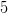. To do that, we can perform the inverse operation; we can subtractfrom both sides: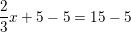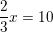Now we have ouron one side and a number on the other, but we need to see what oneis equal to, not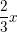. We therefore need to multiplyby a number which will make it just. You might remember from fractions that multiplying a fraction by its reciprocal will give you, so let's try multiplying each side by the reciprocal of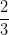, which is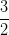: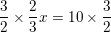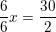We have oneon the left side and a number on the right side, therefore our final answer is### All Basic Arithmetic Resources